# Corresponding labeled objects in two images

Several questions I've seen about bwlabel are about finding the correspondences between object labels in two images. In other words, if a particular pixel is in the foreground in two binary images, is that pixel labeled the same in both images, or is it different? In general, for foreground pixels in the same locations, how do object labels in one binary image match up to object labels in the second?

I'll show one method using the same small binary image that I've been using in my other recent bwlabel posts.

url = 'https://blogs.mathworks.com/images/steve/186/scanned_page.png';
bw = ~bw(1107:1194, 17:135);
imshow(bw, 'InitialMagnification', 'fit')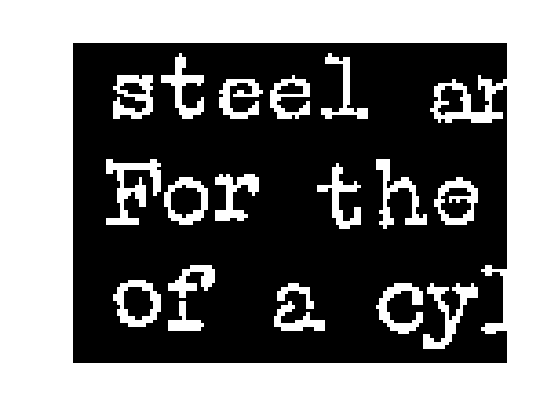Compute and display the labeled objects.

L1 = bwlabel(bw);

I = im2uint8(bw);
I(~bw) = 200;
I(bw) = 240;
s = regionprops(L1, 'extrema');
imshow(I, 'InitialMagnification', 'fit')
hold on
for k = 1:numel(s)
e = s(k).Extrema;
text(e(1,1), e(1,2), sprintf('%d', k));
end
hold off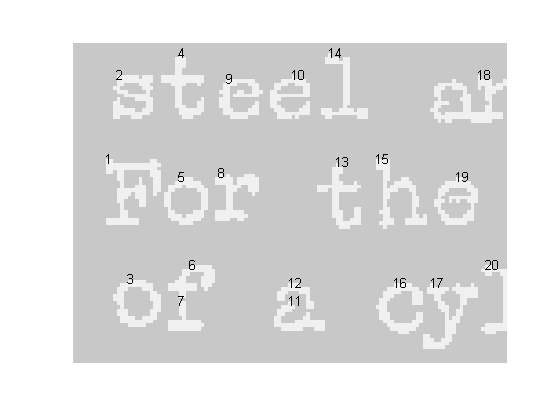Now let's try a morphological closing operation on this image.

bw2 = imclose(bw, ones(3,3));
imshow(bw2, 'InitialMagnification', 'fit')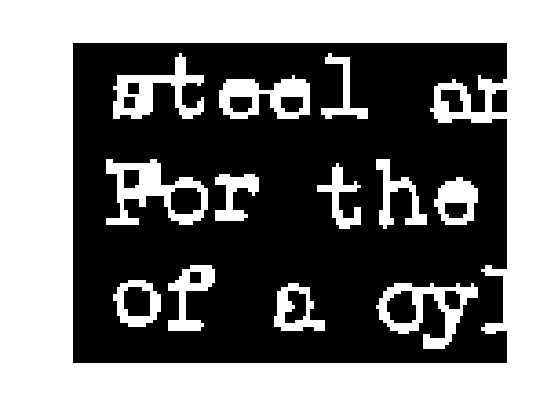Compute and display the labeled objects in the second image.

L2 = bwlabel(bw2);

I2 = im2uint8(bw2);
I2(~bw2) = 200;
I2(bw2) = 240;
s2 = regionprops(L2, 'extrema');
imshow(I2, 'InitialMagnification', 'fit')
hold on
for k = 1:numel(s2)
e2 = s2(k).Extrema;
text(e2(1,1), e2(1,2), sprintf('%d', k));
end
hold off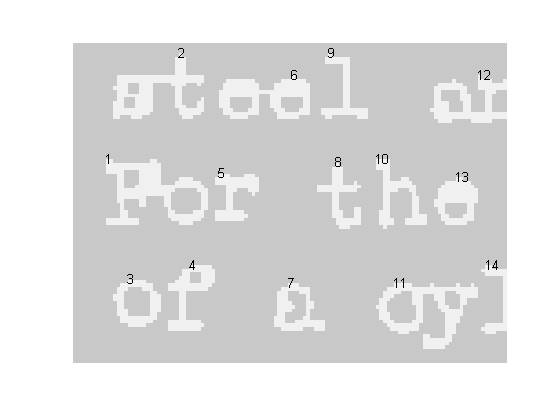Compare the two sets of labels visually. You can see that the broken "f" character on the bottom line was labeled with 6 and 7 in the first image. In the second image, the broken pieces have been merged and labeled with 4.

On the first line of the first image, the "s" and "t" characters are distinct and are labeled with 2 and 4, respectively. In the second image, they have been merged into one object that was labeled 2.

Let's see how to compute all of the correspondences between the object labels in the two images. First, compute the set of pixels that belong to the foreground in both images.

overlap = bw & bw2;

Next, compute the corresponding label pairs by using logical indexing into the two label matrices.

pairs = [L1(overlap), L2(overlap)];

Eliminate the duplicates in the list of pairs.

pairs = unique(pairs, 'rows');

Let's look at a few of the pairs to see how to interpret their meaning:

pairs(1:5, :)
ans =

1     1
2     2
3     3
4     2
5     1



Pixels belonging to objects 1 and 5 in the first image belong to a single object, labeled 1, in the second image. Similarly, pixels belonging to objects 2 and 4 in the first image belong to a single object, labeled 2, in the second image. Pixels labeled with a 3 in the first image are also labeled with a 3 in the second image.

Depending on your application, you might want to form an adjacency graph, represented as a sparse matrix.

S = sparse(pairs(:,1), pairs(:,2), 1);
spy(S)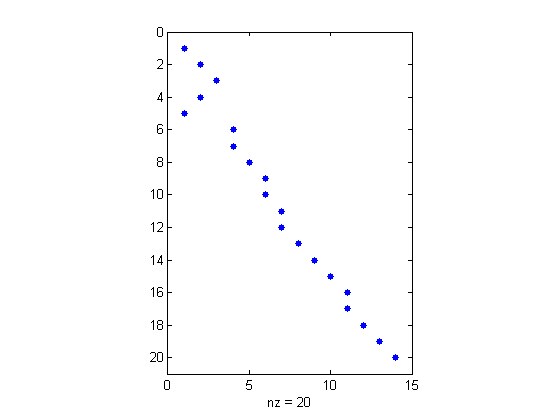One way to use the graph is to find all the object labels in image 1 that correspond to a given label in image 2. For example, here are all the first-image objects corresponding to the object labeled with 6 in the second image:

find(S(:, 6))
ans =

9
10



My other recent bwlabel posts were about search order and relabeling. You can also look the archive of all my posts about connected-component labeling.

Published with MATLAB® 7.6

|